# Calculations of Solution ConcentrationPage 1

#### WATCH ALL SLIDES

Slide 1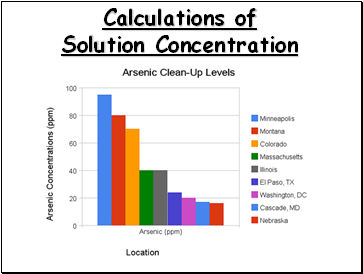Calculations of Solution Concentration

Slide 2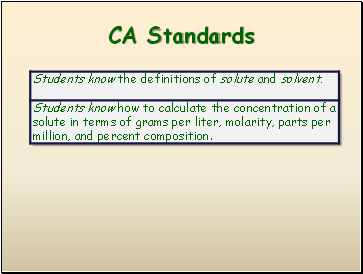## CA Standards

Slide 3## Calculations of Solution Concentration

Grams per Liter

Grams per liter is the ratio of mass units of solute to volume (liters) of solution

Slide 4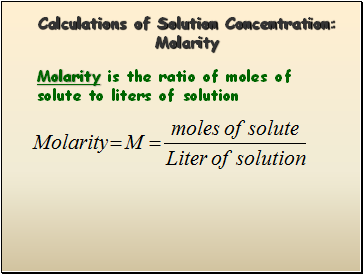Calculations of Solution Concentration: Molarity

Molarity is the ratio of moles of solute to liters of solution

Slide 5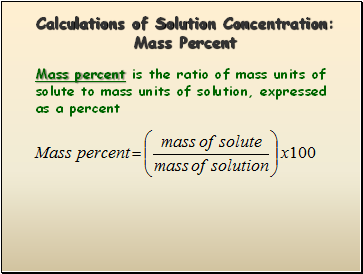Calculations of Solution Concentration: Mass Percent

Mass percent is the ratio of mass units of solute to mass units of solution, expressed as a percent

Slide 6Calculations of Solution Concentration: Parts per Million

Parts per million is the ratio of mass units of solute to mass units of solution, multiplied by one million (106)

Slide 7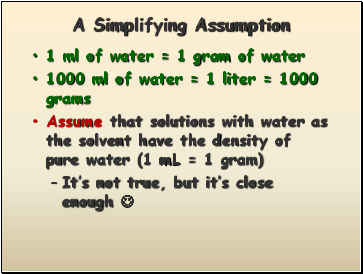## A Simplifying Assumption

1 ml of water = 1 gram of water

1000 ml of water = 1 liter = 1000 grams

Assume that solutions with water as the solvent have the density of pure water (1 mL = 1 gram)

It’s not true, but it’s close enough 